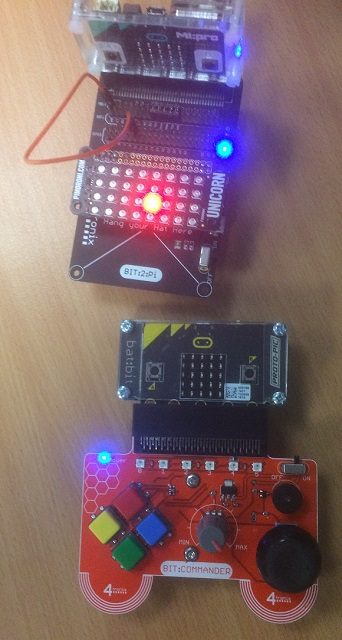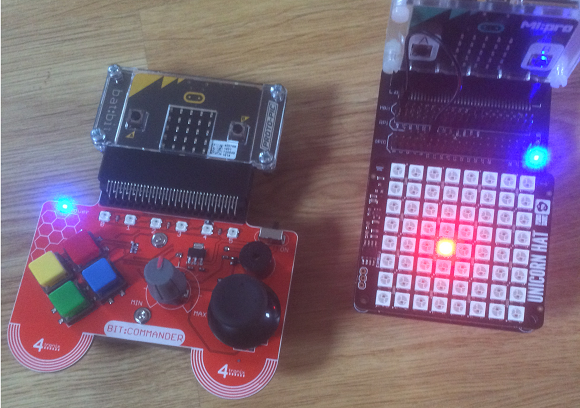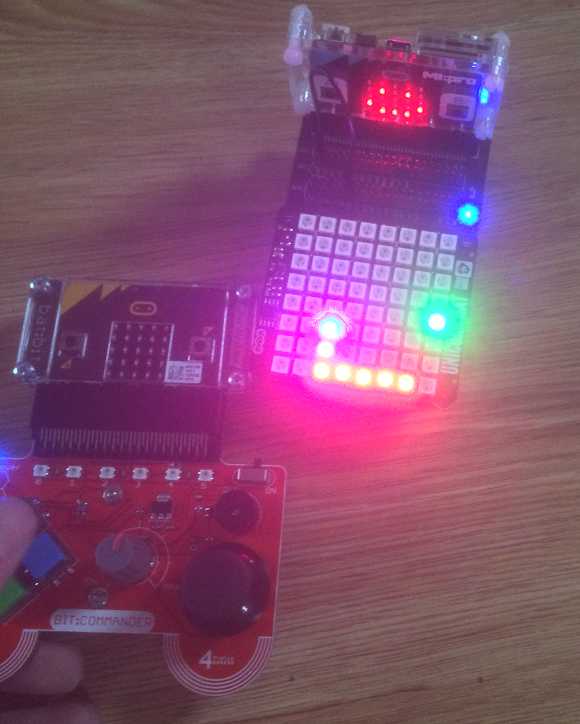# BBC micro:bit Bit:Commander - Unicorn Commander

## Introduction

It's quite easy to knock up a quick project using the Bit:Commander as a controller. When the 5x5 matrix isn't quite enough, you can step up to one of the larger Neopixel matrices and rewrite your matrix games on a larger canvas. For this little project, I used the Unicorn pHAT, a Raspberry Pi accessory made by Pimoroni. I also used the 4tronix Bt:2:Pi.

This is a double micro:bit project. The commands entered on the Bit:Commander are sent by radio to the micro:bit that is connected to the Unicorn pHAT.## Sending

```from microbit import *

chnl = 10

# read the buttons and use binary 4 bits to represent
# the button states
def get_btns():
pattern = 0
for i,p in enumerate([pin12,pin15,pin14,pin16]):
return pattern

last = 0
while True:
btns = get_btns()
e = [((last >> i & 1)<<1) + (btns >> i & 1) for i in range(4)]
if e==2:
elif e==2:
elif e==2:
elif e==2:
last = btns
sleep(20)
```

## Receiving

```from microbit import *
import neopixel

chnl = 10

# Initialise neopixels
npix = neopixel.NeoPixel(pin0, 32)

# Define some colours
red = (64,0,0)
green = (0,64,0)
blue = (0,0,64)
nocol = (0,0,0)

# light all neopixels with given colour
def light_all(col):
for pix in range(0, len(npix)):
npix[pix] = col
npix.show()

# set a pixel colour using x,y coordinates
def set_pix(x,y,col):
npix[y*8+x] = col
npix.show()

# turn them off
light_all(nocol)
sleep(500)
x = 3
y = 1
set_pix(x,y,red)
while True:
if s is not None:
set_pix(x,y,nocol)
if s=="N":
y -= 1
elif s=="S":
y += 1
elif s=="E":
x += 1
elif s=="W":
x -= 1
x = max(0,min(x,7))
y = max(0,min(y,3))
set_pix(x,y,red)
sleep(20)
```

This idea is quite easy to replicate with other Neopixel matrices. The trick is to work out a formula to convert a grid position into a single number representing the position of the pixel in the chain. In this program, the set_pix function does that.When you use a different Neopixel matrix, you first work out how the pixels are connected, then work out a formula to turn a grid position into a pixel number. For the Unicorn HAT (the 8x8 matrix), the pixels are numbered up and down in rows. The following program is the movable dot for the larger matrix.

```from microbit import *
import neopixel

chnl = 10

# Initialise neopixels
npix = neopixel.NeoPixel(pin0, 64)

# Define some colours
red = (32,0,0)
green = (0,32,0)
blue = (0,0,32)
nocol = (0,0,0)

# light all neopixels with given colour
def light_all(col):
for pix in range(0, len(npix)):
npix[pix] = col
npix.show()
return

# wipe a colour across pixels one at a time
def wipe(col, delay):
for pix in range(0, len(npix)):
npix[pix] = col
npix.show()
sleep(delay)
return

# set a pixel colour using x,y coordinates
def set_pix(x,y,col):
if x%2==0:
npix[x*8+y] = col
else:
npix[x*8+7-y] = col
npix.show()

# translate coordinates to pixel number
def xy2pix(x,y):
if x%2==0:
return x*8+y
else:
return x*8+7-y

# turn them off
light_all(nocol)
x = 4
y = 4
set_pix(x,y,red)
while True:
if s is not None:
set_pix(x,y,nocol)
if s=="N":
y -= 1
elif s=="S":
y += 1
elif s=="E":
x += 1
elif s=="W":
x -= 1
x = max(0,min(x,7))
y = max(0,min(y,7))
set_pix(x,y,red)
sleep(20)
```

### Snake

Snake needs a little more space than the built-in matrix allows. The 8x8 grid of the Unicorn HAT is large enough to make the game interesting to play. In the image below, there is a Unicorn HAT mounted on a Bit:2:Pi. The pixels are set at quite low brightness but are still difficult to capture in a photograph.The program receives the same signals from the Bit:Commander. The pushbuttons are used for directional control and to start the game. You might want to change the first example to look for a 1 instead of a 2. That way, button presses, rather than releases, will trigger the movements.

This is a far from perfect way to implement the game of snake but it worked well enough to prove the concept.

```from microbit import *
import neopixel
import random

chnl = 10

# Initialise neopixels
npix = neopixel.NeoPixel(pin0, 64)

# Define some colours
red = (32,0,0)
green = (0,32,0)
blue = (0,0,32)
nocol = (0,0,0)

# light all neopixels with given colour
def light_all(col):
for pix in range(0, len(npix)):
npix[pix] = col

def light_snake(on=True):
hx,hy = snake
if on:
set_pix(hx,hy,blue)
else:
set_pix(hx,hy,nocol)
# light rest of snake red
for i in range(1,len(snake)):
xx,yy = snake[i]
if on:
set_pix(xx,yy,red)
else:
set_pix(xx,yy,nocol)

def update_snake(x,y):
for i in range(len(snake)-1,0,-1):
snake[i] = snake[i-1]
snake = (x,y)

# set a pixel colour using x,y coordinates
def set_pix(x,y,col):
if x%2==0:
npix[x*8+y] = col
else:
npix[x*8+7-y] = col

def place_munch():
p = random.randint(0,63)
while npix[p] != nocol:
p = random.randint(0,63)
return p

# translate coordinates to pixel number
def xy2pix(x,y):
if x%2==0:
return x*8+y
else:
return x*8+7-y

def play_game():
global snake
snake = [(4,4)]
light_snake(True)
munch = place_munch()
npix[munch] = green
npix.show()
dx = -1
dy = 0
delay = 500
last = running_time()
playing = True
while playing:
if s is not None:
if s=="N":
dy = -1
dx = 0
elif s=="S":
dy = 1
dx = 0
elif s=="E":
dx = 1
dy = 0
elif s=="W":
dx = -1
dy = 0
if running_time()-last>delay:
# undraw snake
light_snake(False)
x,y = snake
x = x + dx
y = y + dy
if x<0: x = 7
if x>7: x = 0
if y<0: y = 7
if y>7: y = 0
# check for munch
if xy2pix(x,y)==munch:
snake.append((0,0))
# update snake parts
update_snake(x,y)
munch = place_munch()
npix[munch] = green
elif (x,y) in snake:
# collision with body
playing = False
sleep(3000)
light_all(nocol)
npix.show()
display.show(Image.ARROW_N)
else:
# update snake parts
update_snake(x,y)
light_snake(True)
npix[munch] = green
npix.show()
last = running_time()

light_all(nocol)
npix.show()
snake = [(4,4)]
display.show(Image.ARROW_N)
while True: